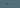# EPSO E-tray scoring the results

November 19th, 2019 - EPSO(1 min)I’ve been struggling for a while how to score my answers to the tests and I’ve come up with two methods:

1. Calculate the deviation
2. Calculate the correctness

## Deviation

The first method calculates the distance from the correct answer.

Table below shows the matrix of scores for Correct vs Selected answers:

C \ S--/++++
01234
-10123
-/+21012
+32101
++43210

To use the table, select the value at the intersection of the row representing the correct answer and the column with your selected answer.

In this variant, ideal score is 0. The maximum possible score (with three options out of 5) is then 11.

## Correctness

Correctness is the opposite of the deviation score; you measure how close you are to the correct answer.

Following table shows the Correct vs Selected answers:

C \ S--/++++
43210
-34321
-/+23432
+12343
++01234

To use it, select the value at the intersection of the row representing the correct answer and the column with your selected answer.

In this case, the maximum possible score you can have in a question (three answers out of five) is 11. The minimum score would be 1.

The values obtained in the two variants are complementary: their sum is always 12.

HTH,

Tagged with
All Tags
Share on

A little experiment: